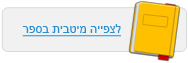עמוד:33 Activity Interactive Simulations Checking the Law of the Conservation of Energy Run the Energy of a Pendulum simulation . Observe the accompanying graphs ( histogram graph and pie chart ) . 1 . This is one of the simulation screens . Let's analyze it : a . What do the blue and yellow sectors represent ? b . What does the entire circle represent ? c . Which of the pendulum positions does the present chart correspond to ? You may express the answer either in words or draw the pendulum in the appropriate position . d . How does the pie diagram illustrate the law of conservation of energy ? e . The pie diagram changes according to the movement of the pendulum . How do the changes in the pie diagram reflect the changes in the pendulum’s energy ? 2 . Observe the column chart in the simulation . It describes the amounts of the pendulum’s kinetic energy and potential energy . The orange color indicates kinetic energy , and the blue indicates potential energy . a . The left column is made up of the other columns , drawn one on top of the other . How does the height of the left column change ? What does this teach us ? b . The following chart describes the amounts of the pendulum’s different energy types at a given moment . Describe the position of the pendulum at this moment ( either in words or using a drawing ) .© מטח - המרכז לטכנולוגיה חינוכית